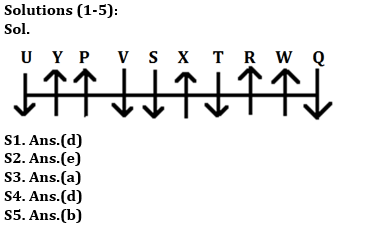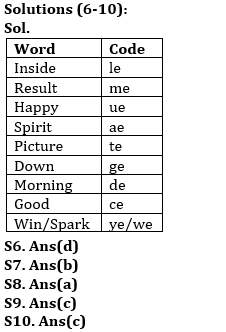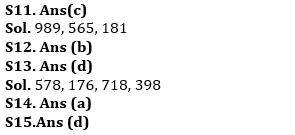Latest Banking jobs   »   Reasoning Ability Quiz For ESIC- UDC,...

# Reasoning Ability Quiz For ESIC- UDC, Steno, MTS Prelims 2022- 20th January

Directions (1-5): Study the information and answer the following questions:
Ten persons are sitting in a row some are facing north, and some are facing south (but not necessarily in the same manner). Not more than two persons facing same direction sits together.
Five persons sit between P and W and both faces same direction. Neither P nor W sits at any end. U sits second to the left of P. Q is an immediate neighbor of W. S sits fourth to the left of U and both faces same direction. T sits third to the right of Q and faces same direction as Q. Y, who faces north is not a neighbor of S and T. X sit second to the left of R and both faces same direction. U is facing south direction. V neither face same direction as R nor is an immediate neighbor of W.

Q1. Who among the following sits to the immediate right to T?
(a) S
(b) W
(c) U
(d) X
(e) None of these

Q2. How many persons sit between Q and V?
(a) One
(b) Four
(c) Two
(d) Three
(e) More than four

Q3. Four of the following five are alike in a certain way and form a group, who among the following does not belongs to that group?
(a) Q
(b) X
(c) Y
(d) W
(e) P

Q4. Which among the following pair sits at the extreme ends of the row?
(a) P, U
(b) S, X
(c) Q, X
(d) U, Q
(e) None of these

Q5. How many persons face south?
(a) Four
(b) Five
(c) Three
(d) One
(e) Two

Directions (6-10): Read the following information carefully and answer the questions given below.
In a certain code language:
“Inside result happy spirit” is written as “ae me le ue”.
“Picture down morning inside” is written as “de le te ge”.
“Happy picture good spirit” is written as “te ae ue ce”.
“Spirit win morning spark” is written as “de ye we ae”.

Q6. What is the possible code for “good way down”?
(a) ce me ge
(b) pe me ge
(c) ce te le
(d) pe ce ge
(e) None of these

Q7. If “Inside win picture” is coded as “ye le te”, then what will be the code for “Spark”?
(a) xe
(b) we
(c) ce
(d) de
(e) Can’t determined

Q8. What is the code for “Morning”?
(a) de
(b) te
(c) ae
(d) ue
(e) None of these

Q9. What is the word for the code “me” in the given code language?
(a) spirit
(b) good
(c) result
(d) spark
(e) win

Q10. If “Spirit give happy” is written as “ue ae re”, then what will be the code for “Give”?
(a) ae
(b) ue
(c) re
(e) Can’t determine

Direction (11-15): Study the following alphanumeric series carefully and answer the questions given below:

4 3 2 5 6 5 7 8 9 8 9 1 7 6 4 2 3 1 5 7 1 8 1 8 9 7 5 3 9 8 4 3 5

Q11. How many such even numbers are there which is immediately preceded as well as immediately followed by same odd number?
(a) One
(b) Two
(c) Three
(d) Four
(e) None

Q12. Which of the following number is 15th from right end of the series?
(a) 1
(b) 5
(c) 3
(d) 7
(e) None of these

Q13. How many such odd numbers are there which is immediately preceded by odd numbers and immediately followed by even numbers?
(a) Two
(b) None
(c) One
(d) Four
(e) More than four

Q14.How many numbers are there in between the number which is 10th from left end and the number which is 14th from right end?
(a) Nine
(b) Ten
(c) Eleven
(d) Eight
(e) None of these

Q15. If all odd numbers are eliminated from the series then, which of the following number is 7th from right end of the series?
(a) 8
(b) 4
(c) 2
(d) 6
(e) None of these

Solutions#### Congratulations!Union Budget 2023-24: Free PDF